# wcoherence

Wavelet coherence and cross-spectrum

## Syntax

``wcoh = wcoherence(x,y)``
``````[wcoh,wcs] = wcoherence(x,y)``````
``````[wcoh,wcs,period] = wcoherence(x,y,ts)``````
`````` [wcoh,wcs,f] = wcoherence(x,y,fs)``````
``````[wcoh,wcs,f,coi] = wcoherence(___)``````
`````` [wcoh,wcs,period,coi] = wcoherence(___,ts)``````
``[___,coi,wtx,wty] = wcoherence(___)``
``[___] = wcoherence(___,Name,Value)``
``wcoherence(___) ``

## Description

example

````wcoh = wcoherence(x,y)` returns the magnitude-squared wavelet coherence, which is a measure of the correlation between signals `x` and `y` in the time-frequency plane. Wavelet coherence is useful for analyzing nonstationary signals. The inputs `x` and `y` must be equal length, 1-D, real-valued signals. The coherence is computed using the analytic Morlet wavelet.```
``````[wcoh,wcs] = wcoherence(x,y)``` returns the wavelet cross-spectrum of `x` and `y`. You can use the phase of the wavelet cross-spectrum values to identify the relative lag between the input signals.```

example

``````[wcoh,wcs,period] = wcoherence(x,y,ts)``` uses the positive `duration` `ts` as the sampling interval. The duration `ts` is used to compute the scale-to-period conversion, `period`. The duration array `period` has the same format as specified in `ts`.```

example

`````` [wcoh,wcs,f] = wcoherence(x,y,fs)``` uses the positive sampling frequency, `fs`, to compute the scale-to-frequency conversion, `f`. The sampling frequency `fs` is in Hz.```
``````[wcoh,wcs,f,coi] = wcoherence(___)``` returns the cone of influence, `coi`, for the wavelet coherence in cycles per sample. If you specify the sampling frequency, `fs`, the cone of influence is in Hz.```

example

`````` [wcoh,wcs,period,coi] = wcoherence(___,ts)``` returns the cone of influence, `coi`, in cycles per unit time. ```
````[___,coi,wtx,wty] = wcoherence(___)` returns the continuous wavelet transforms (CWT) of `x` and `y` in `wtx`, `wty`, respectively. `wtx` and `wty` are used in the formation of the wavelet cross spectrum and coherence estimates.```
````[___] = wcoherence(___,Name,Value)` specifies additional options using one or more name-value pair arguments. This syntax may be used in any of the previous syntaxes.```

example

````wcoherence(___) ` with no output arguments plots the wavelet coherence and cone of influence in the current figure. Due to the inverse relationship between frequency and period, a plot that uses the sampling interval is the inverse of a plot the uses the sampling frequency. For areas where the coherence exceeds 0.5, plots that use the sampling frequency display arrows to show the phase lag of `y` with respect to `x`. The arrows are spaced in time and scale. The direction of the arrows corresponds to the phase lag on the unit circle. For example, a vertical arrow indicates a π/2 or quarter-cycle phase lag. The corresponding lag in time depends on the duration of the cycle.```

## Examples

collapse all

Use default `wcoherence` settings to obtain the wavelet coherence between a sine wave with random noise and a frequency-modulated signal with decreasing frequency over time.

```t = linspace(0,1,1024); x = -sin(8*pi*t) + 0.4*randn(1,1024); x = x/max(abs(x)); y = wnoise('doppler',10); wcoh = wcoherence(x,y);```

The default coherence computation uses the analytic Morlet wavelet, 12 voices per octave and smooths 12 scales. The default number of octaves is equal to `floor(log2(numel(x)))-1`, which in this case is 9.

Obtain the wavelet coherence data for two signals, specifying a sampling interval of 0.001 seconds. Both signals consist of two sine waves (10 Hz and 50 Hz) in white noise. The sine waves have different time supports.

Set the random number generator to its default settings for reproducibility. Then create the two signals.

```rng default; t = 0:0.001:2; x = cos(2*pi*10*t).*(t>=0.5 & t<1.1)+ ... cos(2*pi*50*t).*(t>= 0.2 & t< 1.4)+0.25*randn(size(t)); y = sin(2*pi*10*t).*(t>=0.6 & t<1.2)+... sin(2*pi*50*t).*(t>= 0.4 & t<1.6)+ 0.35*randn(size(t)); subplot(2,1,1) plot(t,x) title('X') subplot(2,1,2) plot(t,y) title('Y') xlabel('Time (seconds)')```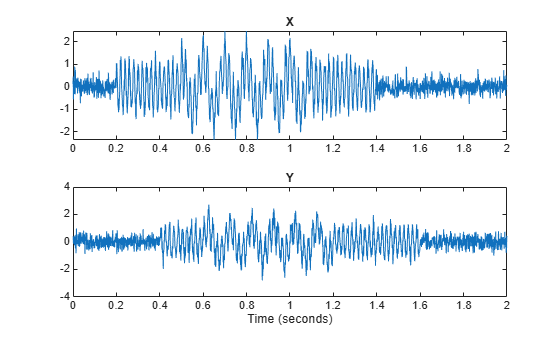Obtain the coherence of the two signals.

`[wcoh,~,period,coi] = wcoherence(x,y,seconds(0.001));`

Use the `pcolor` command to plot the coherence and cone of influence.

```figure period = seconds(period); coi = seconds(coi); h = pcolor(t,log2(period),wcoh); h.EdgeColor = 'none'; ax = gca; ytick=round(pow2(ax.YTick),3); ax.YTickLabel=ytick; ax.XLabel.String='Time'; ax.YLabel.String='Period'; ax.Title.String = 'Wavelet Coherence'; hcol = colorbar; hcol.Label.String = 'Magnitude-Squared Coherence'; hold on; plot(ax,t,log2(coi),'w--','linewidth',2)```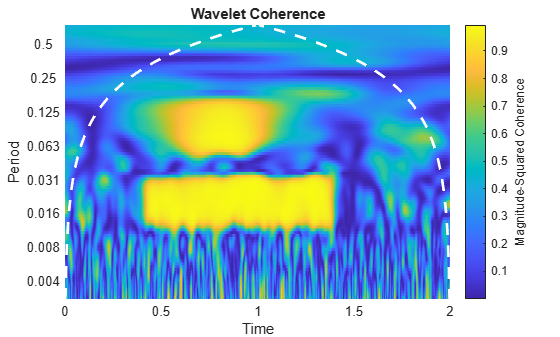Use `wcoherence(x,y,seconds(0.001))` without any outputs arguments. This plot includes the phase arrows and the cone of influence.

`wcoherence(x,y,seconds(0.001));`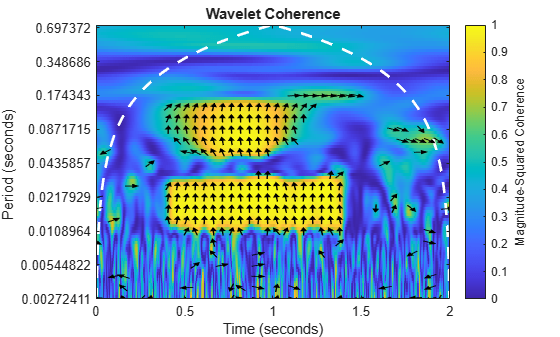Obtain the wavelet coherence for two signals, specifying a sampling frequency of 1000 Hz. Both signals consist of two sine waves (10 Hz and 50 Hz) in white noise. The sine waves have different time supports.

Set the random number generator to its default settings for reproducibility and create the two signals.

```rng default t = 0:0.001:2; x = cos(2*pi*10*t).*(t>=0.5 & t<1.1)+... cos(2*pi*50*t).*(t>= 0.2 & t< 1.4)+0.25*randn(size(t)); y = sin(2*pi*10*t).*(t>=0.6 & t<1.2)+... sin(2*pi*50*t).*(t>= 0.4 & t<1.6)+ 0.35*randn(size(t));```

Obtain the wavelet coherence. The coherence plot is flipped with respect to the plot in the previous example, which specifies a sampling interval instead of a sampling frequency.

`wcoherence(x,y,1000)`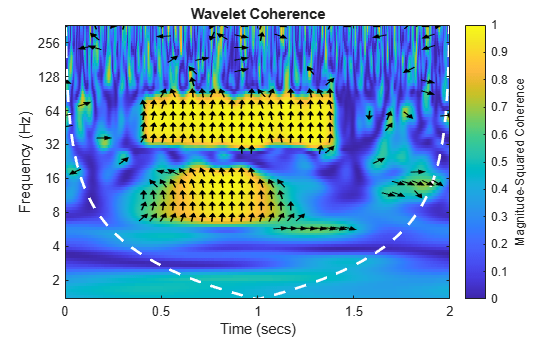Obtain the scale-to-frequency conversion output in `f`.

`[wcoh,wcs,f] = wcoherence(x,y,1000);`

Obtain the wavelet coherence for two signals. Both signals consist of two sine waves (10 Hz and 50 Hz) in white noise. Use the default number of scales to smooth. This value is equivalent to the number of voices per octave. Both values default to 12.

Set the random number generator to its default settings for reproducibility. Then, create the two signals and obtain the coherence.

```rng default; t = 0:0.001:2; x = cos(2*pi*10*t).*(t>=0.5 & t<1.1)+ ... cos(2*pi*50*t).*(t>= 0.2 & t< 1.4)+0.25*randn(size(t)); y = sin(2*pi*10*t).*(t>=0.6 & t<1.2)+... sin(2*pi*50*t).*(t>= 0.4 & t<1.6)+ 0.35*randn(size(t)); wcoherence(x,y)```Set the number of scales to smooth to 18. The increased smoothing causes reduced low frequency resolution.

`wcoherence(x,y,'NumScalesToSmooth',18)`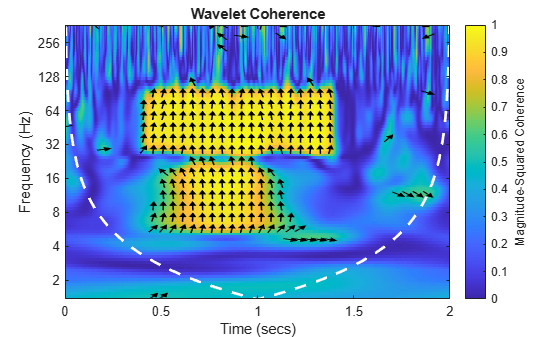Compare the effects of using different phase display thresholds on the wavelet coherence.

Plot the wavelet coherence between the El Nino time series and the All India Average Rainfall Index. The data are sampled monthly. Specify the sampling interval as 1/12 of a year to display the periods in years. Use the default phase display threshold of 0.5, which shows phase arrows only where the coherence is greater than or equal to 0.5.

```load ninoairdata; wcoherence(nino,air,years(1/12));```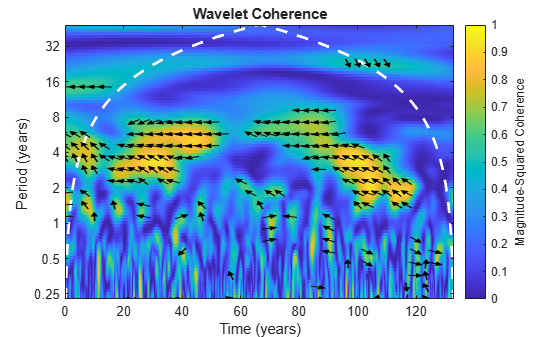Set the phase display threshold to 0.7. The number of phase arrows decreases.

`wcoherence(nino,air,years(1/12),'PhaseDisplayThreshold',0.7);`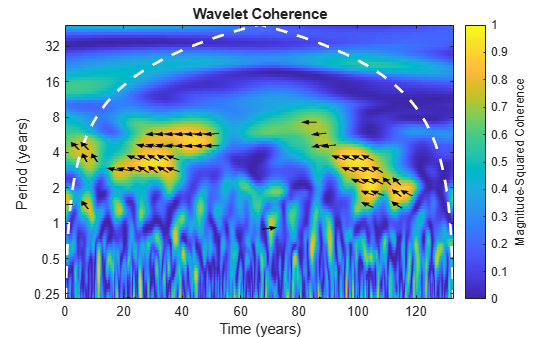## Input Arguments

collapse all

Input signal, specified as a vector of real values. `x` must be a 1-D, real-valued signal. The two input signals, `x` and `y`, must be the same length and must have at least four samples.

Input signal, specified as vector of real values. `y` must be a 1-D, real-valued signal. The two input signals, `x` and `y`, must be the same length and must have at least four samples.

Sampling interval, also known as the sampling period, specified as a `duration` with positive scalar input. Valid durations are `years`, `days`, `hours`, `seconds`, and `minutes`. You can also use the `duration` function to specify `ts`. You cannot use calendar durations (`caldays`, `calweeks`, `calmonths`, `calquarters`, or `calyears`).

You cannot specify both a sampling frequency `fs` and a sampling period `ts`.

Sampling frequency, specified as a positive scalar.

If you specify `fs` as empty, `wcoherence` uses normalized frequency in cycles/sample. The Nyquist frequency is ½.

You cannot specify both a sampling frequency `fs` and a sampling period `ts`.

### Name-Value Pair Arguments

Specify optional comma-separated pairs of `Name,Value` arguments. `Name` is the argument name and `Value` is the corresponding value. `Name` must appear inside quotes. You can specify several name and value pair arguments in any order as `Name1,Value1,...,NameN,ValueN`.

Example: `'PhaseDisplayThreshold',0.7;` specifies the threshold for displaying phase vectors.

Frequency limits to use in `wcoherence`, specified as a two-element vector with positive strictly increasing elements. The first element specifies the lowest peak passband frequency and must be greater than or equal to the product of the wavelet peak frequency in hertz and two time standard deviations divided by the signal length. The second element specifies the highest peak passband frequency and must be less than or equal to the Nyquist frequency. The base 2 logarithm of the ratio of the maximum frequency to the minimum frequency must be greater than or equal to 1/NV where NV is the number of voices per octave.

If you specify frequency limits outside the permissible range, `wcoherence` truncates the limits to the minimum and maximum valid values. Use `cwtfreqbounds` with the wavelet set to `'amor'` to determine frequency limits for different parameterizations of the wavelet coherence.

Example: `'FrequencyLimits',[0.1 0.3]`

Period limits to use in `wcoherence`, specified as a two-element duration array with strictly increasing positive elements. The first element must be greater than or equal to 2×`ts` where `ts` is the sampling period. The base 2 logarithm of the ratio of the minimum period to the maximum period must be less than or equal to -1/NV where NV is the number of voices per octave. The maximum period cannot exceed the signal length divided by the product of two time standard deviations of the wavelet and the wavelet peak frequency.

If you specify period limits outside the permissible range, `wcoherence` truncates the limits to the minimum and maximum valid values. Use `cwtfreqbounds` with the wavelet set to `'amor'` to determine period limits for different parameterizations of the wavelet coherence.

Example: `'PeriodLimits',[seconds(0.2) seconds(1)]`

Data Types: `duration`

Number of voices per octave to use in the wavelet coherence, specified as an even integer from 10 to 32.

Number of scales to smooth in time and scale, specified as a positive integer less than or equal to one half N, where N is the number of scales in the wavelet transform. If unspecified, `NumScalesToSmooth` defaults to the minimum of `floor(N/2)` and `VoicesPerOctave`. The function uses a moving average filter to smooth across scale. If your coherence is noisy, you can specify a larger `NumScalesToSmooth` value to smooth the coherence more.

Number of octaves to use in the wavelet coherence, specified as a positive integer between 1 and `floor(log2(numel(x)))-1`. If you do not need to examine lower frequency values, use a smaller `NumOctaves` value.

The `'NumOctaves'` name-value pair is not recommended and will be removed in a future release. The recommended way to modify the frequency or period range of wavelet coherence is with the `'FrequencyLimits'` or `'PeriodLimits'` name-value pairs. You cannot specify both the `'NumOctaves'` and `'FrequencyLimits'` or `'PeriodLimits'` name-value pairs. See `cwtfreqbounds`.

Threshold for displaying phase vectors, specified as a real scalar between 0 and 1. This function displays phase vectors for regions with coherence greater than or equal to the specified threshold value. Lowering the threshold value displays more phase vectors. If you use `wcoherence` with any output arguments, the `PhaseDisplayThreshold` value is ignored.

## Output Arguments

collapse all

Wavelet coherence, returned as a matrix. The coherence is computed using the analytic Morlet wavelet over logarithmic scales, with a default value of 12 voices per octave. The default number of octaves is equal to `floor(log2(numel(x)))-1`. If you do not specify a sampling interval, sampling frequency is assumed.

Wavelet cross-spectrum, returned as a matrix of complex values. You can use the phase of the wavelet cross-spectrum values to identify the relative lag between the input signals.

Scale-to-period conversion, returned as an array of durations. The conversion values are computed from the sampling period specified in `ts`. Each `period` element has the same format as `ts`.

Scale-to-frequency conversion, returned as a vector. The vector contains the peak frequency values for the wavelets used to compute the coherence. If you want to output `f`, but do not specify a sampling frequency input, `fs`, the returned wavelet coherence is in cycles per sample.

Cone of influence for the wavelet coherence, returned as either an array of doubles or array of durations. The cone of influence indicates where edge effects occur in the coherence data. If you specify a sampling frequency, `fs`, the cone of influence is in Hz. If you specify a sampling interval or period, `ts`, the cone of influence is in periods. Due to the edge effects, give less credence to areas of apparent high coherence that are outside or overlap the cone of influence. The cone of influence is indicated by a dashed line.

For additional information, see Boundary Effects and the Cone of Influence.

Continuous wavelet transform of `x`, returned as a matrix.

Continuous wavelet transform of `y`, returned as a matrix.

collapse all

### Wavelet Cross Spectrum

The wavelet cross-spectrum is a measure of the distribution of power of two signals.

The wavelet cross spectrum of two time series, x and y, is:

`${C}_{xy}\left(a,b\right)=S\left({C}_{x}^{*}\left(a,b\right){C}_{y}\left(a,b\right)\right)$`

Cx(a,b) and Cy(a,b) denote the continuous wavelet transforms of x and y at scales a and positions b. The superscript * is the complex conjugate, and S is a smoothing operator in time and scale.

For real-valued time series, the wavelet cross-spectrum is real-valued if you use a real-valued analyzing wavelet, and complex-valued if you use a complex-valued analyzing wavelet.

### Wavelet Coherence

Wavelet coherence is a measure of the correlation between two signals.

The wavelet coherence of two time series x and y is:

`$\frac{{|S\left({C}_{x}^{*}\left(a,b\right){C}_{y}\left(a,b\right)\right)|}^{2}}{S\left(|{C}_{x}\left(a,b\right){|}^{2}\right)·S\left(|{C}_{y}\left(a,b\right){|}^{2}\right)}$`

Cx(a,b) and Cy(a,b) denote the continuous wavelet transforms of x and y at scales a and positions b. The superscript * is the complex conjugate and S is a smoothing operator in time and scale.

For real-valued time series, the wavelet coherence is real-valued if you use a real-valued analyzing wavelet, and complex-valued if you use a complex-valued analyzing wavelet.

## Compatibility Considerations

expand all

Not recommended starting in R2020a

 Grinsted, A, J., C. Moore, and S. Jevrejeva. “Application of the cross wavelet transform and wavelet coherence to geophysical time series.” Nonlinear Processes in Geophysics. Vol. 11, Issue 5/6, 2004, pp. 561–566.

 Maraun, D., J. Kurths, and M. Holschneider. "Nonstationary Gaussian processes in wavelet domain: Synthesis, estimation and significance testing.” Physical Review E 75. 2007, pp. 016707-1–016707-14.

 Torrence, C., and P. Webster. "Interdecadal changes in the ESNO-Monsoon System." Journal of Climate. Vol. 12, 1999, pp. 2679–2690.

## SupportGet trial now